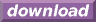#Calcute: freeware calculator

## Arccotangent (arc-cotangent, inverse cotangent, acot)

The arc-cotangent is the inverse of the cotangent function. Calcute implements it with the acot function. The inverse cotangent of a value x is the value y for which the cotangent of y is x. In other words if y = acot(x) then x = cot(y). And since the cotangent takes an angle as input parameter, the arccotangent produces an angle as its output.

```acot(1)
0.785398163397
```

The acot value is expressed by Calcute using the currently-selected angle unit: radian, degree or gradient. In the above example, the result is in radians.

Note that the cotangent function is periodic: its values repeat infinitely for angles that represent more than half of one complete rotation. Conversely, the arccotangent is a multi-valued function: any parameter value matches an infinite number of possible angles. But the acot function generates a single value refered to as the principal value. This is the "nearest" valid angle that remains within half of one revolution. All other possibilities can be obtained by adding or subtracting any whole number of half rotations from the return value of acot.

In some mathematical texts and hand-held calculators, the notation cot-1 is sometimes used to represent the arc-cotangent function. This is an unfortunate notational choice since it could also stand for the multiplicative inverse 1/cot, which is a different function.

Yet another term also used to represent the inverse cotangent function is "arccot".